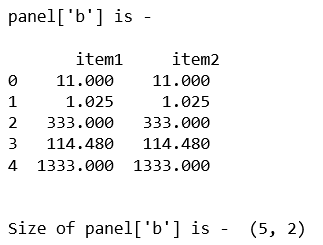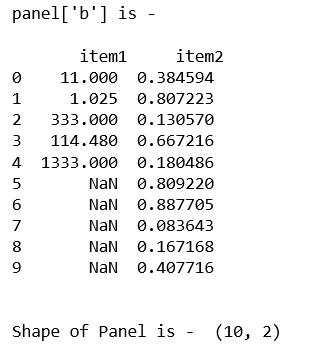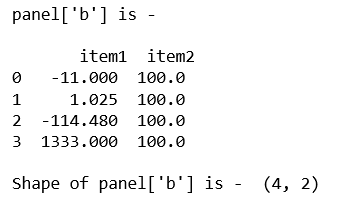# Python | Pandas Panel.shape

• Last Updated : 30 Jun, 2021

In Pandas, Panel is a very important container for three-dimensional data. The names for the 3 axes are intended to give some semantic meaning to describing operations involving panel data and, in particular, econometric analysis of panel data.
In Pandas Panel.shape can be used to get a tuple of axis dimensions.

Syntax: Panel.shape
Parameters: None
Returns: Return a tuple of axis dimensions

Code #1:

## Python3

 `# importing pandas module ``import` `pandas as pd ``import` `numpy as np``  ` `df1 ``=` `pd.DataFrame({``'a'``: [``'Geeks'``, ``'For'``, ``'geeks'``, ``'for'``, ``'real'``], ``                    ``'b'``: [``11``, ``1.025``, ``333``, ``114.48``, ``1333``]})``                      ` `data ``=` `{``'item1'``:df1, ``'item2'``:df1}``  ` `# creating Panel ``panel ``=` `pd.Panel.from_dict(data, orient ``=``'minor'``)``  ` `print``(``"panel['b'] is - \n\n"``, panel[``'b'``], ``'\n'``)``  ` `print``(``"\nSize of panel['b'] is - "``, panel[``'b'``].shape)  `

Output:Code #2:

## Python3

 `# importing pandas module ``import` `pandas as pd ``import` `numpy as np``  ` `df1 ``=` `pd.DataFrame({``'a'``: [``'Geeks'``, ``'For'``, ``'geeks'``, ``'for'``, ``'real'``], ``                    ``'b'``: [``11``, ``1.025``, ``333``, ``114.48``, ``1333``]})` `# Create a 5 * 5 dataframe``df2 ``=` `pd.DataFrame(np.random.rand(``10``, ``2``), columns ``=``[``'a'``, ``'b'``])``  ` `data ``=` `{``'item1'``:df1, ``'item2'``:df2}``  ` `# creating Panel ``panel ``=` `pd.Panel.from_dict(data, orient ``=``'minor'``)``print``(``"panel['b'] is - \n\n"``, panel[``'b'``], ``'\n'``)`  `print``(``"\nShape of Panel is - "``, panel[``'b'``].shape)`

Output:Code #3:

## Python3

 `# importing pandas module``import` `pandas as pd``import` `numpy as np` `df1 ``=` `pd.DataFrame({``'a'``: [``'Geeks'``, ``'For'``, ``'geeks'``, ``'real'``],``                    ``'b'``: [``-``11``, ``+``1.025``, ``-``114.48``, ``1333``]})` `df2 ``=` `pd.DataFrame({``'a'``: [``'I'``, ``'am'``, ``'dataframe'``, ``'two'``],``                    ``'b'``: [``100``, ``100``, ``100``, ``100``]})``                    ` `data ``=` `{``'item1'``:df1, ``'item2'``:df2}` `# creating Panel``panel ``=` `pd.Panel.from_dict(data, orient ``=``'minor'``)``print``(``"panel['b'] is - \n\n"``, panel[``'b'``])` `print``(``"\nShape of panel['b'] is - "``,  panel[``'b'``].shape)`

Output:Note: The panel has been removed from Pandas module 0.25.0 onwards.

## Python3

 `#To check the version of pandas library``import` `pandas``print``(pandas.__version__)`

My Personal Notes arrow_drop_up